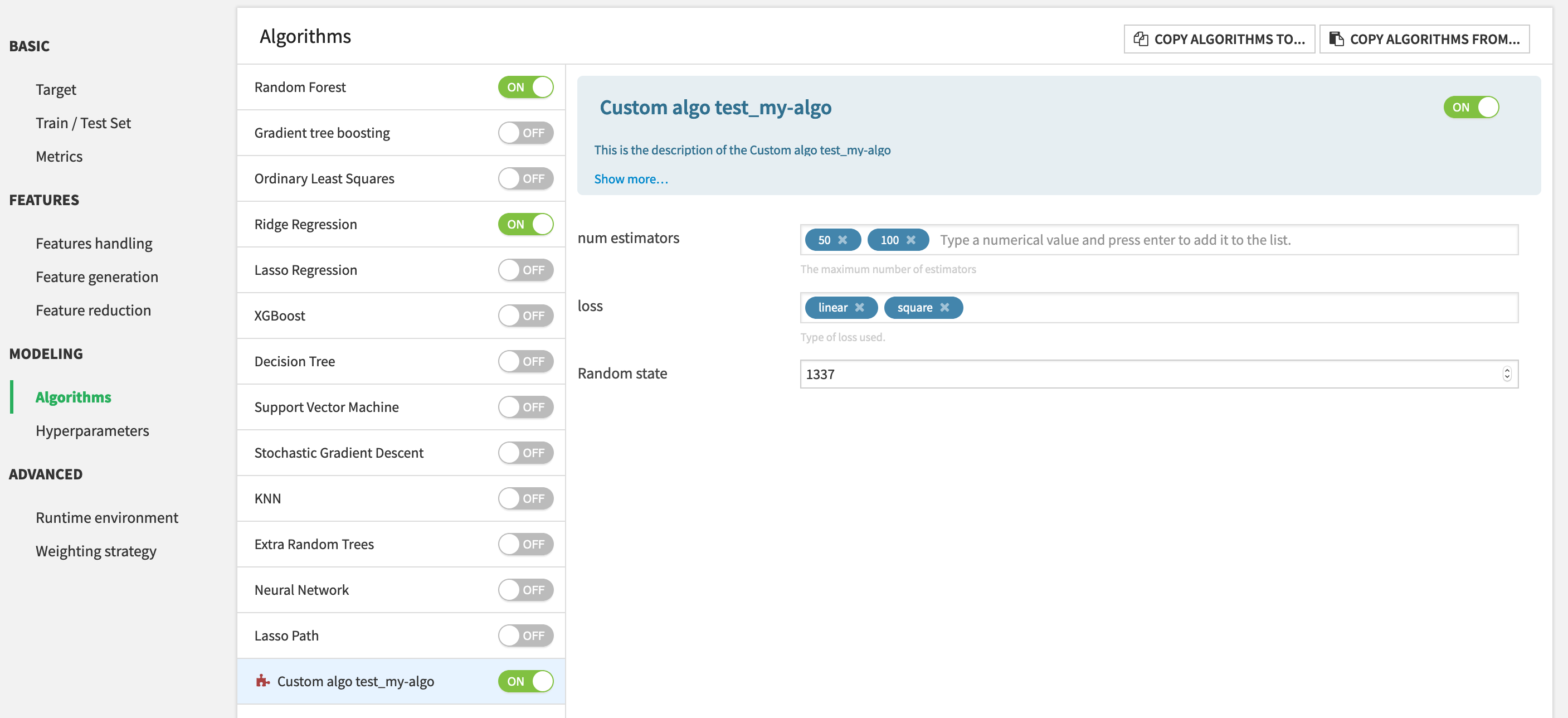# Component: Prediction algorithm¶

Dataiku DSS offers a variety of algorithms to address prediction problems.

This plugin component allows to extend the list of available algorithms, in order to help data scientists expose their custom algorithms to other users.

## First example¶

To start adding prediction algorithms, we recommend that you use the plugin developer tools (see the tutorial for an introduction). In the Definition tab, click on “+ NEW COMPONENT”, choose ”Prediction Algorithms”, and enter the identifier for your new algorithm. You’ll see a new folder `python-prediction-algos` and will have to edit:

• the `algo.json` file, containing the various parameters of your algo

• the `algo.py` file, containing the code of your algorithm

A basic prediction algorithm’s description to add the scikit learn AdaboostRegressor algorithm looks like this:

```{
"meta" : {
"label": "Custom algo test_my-algo",
"description": "This is the description of the Custom algo test_my-algo",
"icon": "icon-puzzle-piece"
},

"predictionTypes": ["REGRESSION"],
"gridSearchMode": "MANAGED",
"supportsSampleWeights": true,
"acceptsSparseMatrix": false,

"params": [
{
"name": "n_estimators",
"label": "num estimators",
"description": "The maximum number of estimators",
"type": "DOUBLES",
"defaultValue": [50, 100],
"allowDuplicates": false,
"gridParam": true
},
{
"name": "loss",
"label": "loss",
"description": "Type of loss used.",
"type": "MULTISELECT",
"defaultValue": ["linear"],
"selectChoices": [
{
"value":"linear",
"label":"linear"
},
{
"value":"square",
"label":"square"
},
{
"value":"exponential",
"label": "exponential"
}
],
"gridParam": true
},
{
"name": "random_state",
"label": "Random state",
"type": "DOUBLE",
"defaultValue": 1337
}
]
}
```

and the corresponding python code:

```from dataiku.doctor.plugins.custom_prediction_algorithm import BaseCustomPredictionAlgorithm

class CustomPredictionAlgorithm(BaseCustomPredictionAlgorithm):
"""
Args:
prediction_type (str): type of prediction for which the algorithm is used. Is relevant when
algorithm works for more than one type of prediction.
params (dict): dictionary of params set by the user in the UI.
"""

def __init__(self, prediction_type=None, params=None):
super(CustomPredictionAlgorithm, self).__init__(prediction_type, params)

def get_clf(self):
"""
This method must return a scikit-learn compatible model, ie:
- have a fit(X,y) and predict(X) methods. If sample weights
are enabled for this algorithm (in algo.json), the fit method
must have instead the signature fit(X, y, sample_weight=None)
- have a get_params() and set_params(**params) methods
"""
return self.clf
```

Once the plugin component is added, it will be available in the visual ML Lab, as any other algorithm.## Algorithm description¶

Each algorithm description has the following structure:

• `predictionTypes`: List of types of prediction for which the algorithm will be available. Possible values are: [“BINARY_CLASSIFICATION”, “MULTICLASS”, “REGRESSION”].

• `gridSearchMode`: How DSS handles gridsearch. See Grid search.

• `supportsSampleWeights`: Whether the model supports or not sample weights for training. If yes, the clf from algo.py must have a `fit(X, y, sample_weights=None)` method. If not, sample weights are not applied on this algorithm, but if they are selected for training, they will be applied on scoring metrics and charts.

• `acceptsSparseMatrix`: Whether the model supports sparse matrices for fitting and predicting, i.e. if the `clf` provided in algo.py accepts a sparse matrix as argument for its `fit` and `predict` methods.

• `params`: List of plugin parameters that can be leveraged by the model, and potentially the grid search (See Grid search).

• `meta`: Description of the component. Similar to other plugin components.

## Algorithm python object¶

The `BaseCustomPredictionAlgorithm` defines a `get_clf` method that must return a scikit-learn compatible object. This means that the model must:

• have `fit(X,y)` and `predict(X)` methods. If sample weights are enabled for this algorithm (in `algo.json`), the fit method must have instead the signature `fit(X, y, sample_weight=None)`.

• have `get_params()` and `set_params(**params)` methods.

Moreover, the model can implement a function called `set_column_labels(self, column_labels)` if it needs to have access to the column names of the preprocessed dataset.

For further information, please refer to Custom Models.

## Usage of a code-env¶

DSS allows the user to create and use code environments to be able to leverage external packages.

Plugin algorithms cannot use the plugin code-env.

If the algorithm code relies on external libraries, a dedicated code-env must be created on the Dataiku DSS instance on which the plugin is installed. This code env must contain both:

• the packages required for Visual Machine Learning

• the packages required for your algorithm

This code env must then be manually selected by the end-user running this plugin algorithm.